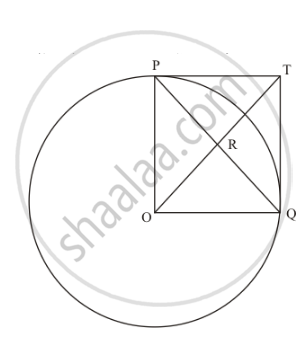Advertisement Remove all ads

# In the Given Figure, Po ⊥ Qo. the Tangents to the Circle at P and Q Intersect at a Point T. Prove that Pq and Otare Right Bisector of Each Other. - Mathematics

Short Note

In the given figure, PO $\perp$  QO. The tangents to the circle at P and Q intersect at a point T. Prove that PQ and OTare right bisector of each other.Advertisement Remove all ads

#### Solution

In the given figure,PO = OQ (Since they are the radii of the same circle)

PT = TQ (Length of the tangents from an external point to the circle will be equal) Now considering the angles of the quadrilateral PTQO, we have,

∠POQ=90^o (Given in the problem)

∠OPT=90^o (The radius of the circle will be perpendicular to the tangent at the point of contact)

∠ TQO=90^o (The radius of the circle will be perpendicular to the tangent at the point of contact)

We know that the sum of all angles of a quadrilateral will be equal to 360^o. Therefore,

∠ POQ+∠TQO+∠OPT+∠PTQ=360^o

90^O+90^O+90^O+∠ PTQ=360^o

∠ PTQ=90^o

Thus we have found that all angles of the quadrilateral are equal to 90°.

Since all angles of the quadrilateral PTQO are equal to 90° and the adjacent sides are equal, this quadrilateral is a square.

We know that in a square, the diagonals will bisect each other at right angles.

Therefore, PQ and OT bisect each other at right angles.

Thus we have proved.

Is there an error in this question or solution?
Advertisement Remove all ads

#### APPEARS IN

RD Sharma Class 10 Maths
Chapter 8 Circles
Exercise 8.2 | Q 47 | Page 40
Advertisement Remove all ads
Advertisement Remove all ads
Share
Notifications

View all notifications

Forgot password?